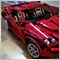# Indicator values only for the last X bars173

Hi there!

Can someone show me how should I modify the original MACD code if I only want the values for the last 100 bars?

I mean, I don't want the indicator to calculate for all candles, only for the last 100 (99-0 index).

Please provide me the modification of this original code:

```int OnCalculate (const int rates_total,
const int prev_calculated,
const datetime& time[],
const double& open[],
const double& high[],
const double& low[],
const double& close[],
const long& tick_volume[],
const long& volume[],
{
int i,limit;
//---
if(rates_total<=InpSignalSMA || !ExtParameters)
return(0);
//--- last counted bar will be recounted
limit=rates_total-prev_calculated;  // I changed this line this way: limit=MathMin(rates_total-prev_calculated, 100); but it gave a weird curve on the chart
if(prev_calculated>0)
limit++;
//--- macd counted in the 1-st buffer
for(i=0; i<limit; i++)
ExtMacdBuffer[i]=iMA(NULL,0,InpFastEMA,0,MODE_EMA,PRICE_CLOSE,i)-
iMA(NULL,0,InpSlowEMA,0,MODE_EMA,PRICE_CLOSE,i);
//--- signal line counted in the 2-nd buffer
SimpleMAOnBuffer(rates_total,prev_calculated,0,InpSignalSMA,ExtMacdBuffer,ExtSignalBuffer);
//--- done
return(rates_total);
}```1216

linux80s:

Hi there!

Can someone show me how should I modify the original MACD code if I only want the values for the last 100 bars?

I mean, I don't want the indicator to calculate for all candles, only for the last 100 (99-0 index).

Please provide me the modification of this original code:

If I ever need to do that, I'll do this:

```input int MaxBars=100;

int OnInit(void)
{
:
SetIndexEmptyValue(1,0);
:
}

int OnCalculate (const int rates_total,
:
{
:
for(i=0; i<limit; i++)
{
if (i>MaxBars)
continue;
ExtMacdBuffer[i]=iMA(NULL,0,InpFastEMA,0,MODE_EMA,PRICE_CLOSE,i)-
iMA(NULL,0,InpSlowEMA,0,MODE_EMA,PRICE_CLOSE,i);
}
//--- signal line counted in the 2-nd buffer
SimpleMAOnBuffer(rates_total,prev_calculated,rates_total-MaxBars,InpSignalSMA,ExtMacdBuffer,ExtSignalBuffer);
:
}

```173

Seng Joo Thio:

If I ever need to do that, I'll do this:

Thank you, this solution works (but instead of continue it is better to use break).

I would need one more thing: your code draws not only the last 100 values, but if a new candle is coming, it will add to the existing ones, so there will be 101, 102 , 103 etc.

But I only need 100, I don't want it to be increased. How?20233

linux80s: But I only need 100, I don't want it to be increased. How?

Don't know why you care so much - just ignore old values. But if you insist, place empty values in the 101 buffers.173

William Roeder:

Don't know why you care so much - just ignore old values. But if you insist, place empty values in the 101 buffers.

Thank you1216

linux80s:

Thank you, this solution works (but instead of continue it is better to use break).

I would need one more thing: your code draws not only the last 100 values, but if a new candle is coming, it will add to the existing ones, so there will be 101, 102 , 103 etc.

But I only need 100, I don't want it to be increased. How?

It draws, but it does not compute. Computation was done once, then the bars are simply "shifted" back. In subsequent bars, only bars 1 and 0 gets updated when new tick comes. So if you insist on setting bars beyond 100 to be zero, you're incurring extra processing by setting them to zero... because the system will still do the "shift" and draw, regardless whether the bars are zero or not.

So yes, you can set bar 101 to zero, as @William Roeder suggested, only if it is aesthetically important to you.